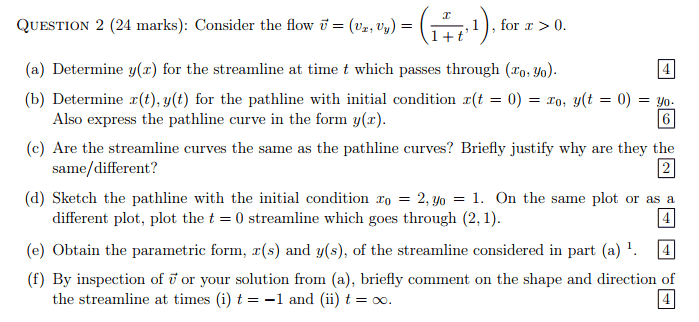# Determining streamlines

## Homework Statement## Homework Equations

$$\frac{dy}{dx}=\frac{v_y}{v_x}$$[/B]

## The Attempt at a Solution

I have subbed the given values for $$v_y$$ $$v_x$$ into the equation above, and integrated, i got the following expression
$$y=\left(1+t \right) lnx$$
I'm not sure where to go next, do I sub in $$x_0$$ $$y_0$$ ?

#### Attachments

Last edited by a moderator:

Orodruin
Staff Emeritus
$$\frac{dx}{dt}= v_x, \quad \frac{dy}{dt}= v_y.$$